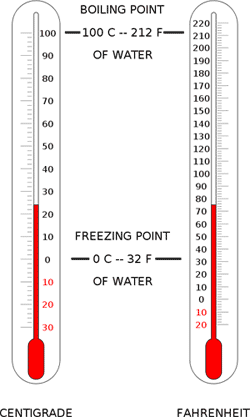# Celsius to Farenheit Conversion

Making Celsius to Fahrenheit conversions is very easy.The following information will show how simple the process actually is.

### How to Convert Temperature from Celsius to Fahrenheit

Step 1

Choose a specific Celsius temperature to convert (i.e., 10 C, 39 C etc).

Step 2

Multiply that Celsius temperature by 9. Divide the result by 5. Add 32 to the result. The result is the temperature in Fahrenheit. The following is an example: 10 degrees C x 9 = 90; 90 / 5 = 18; 18 + 32 = 50. This means 10 degrees C is equal to 50 degrees F.

Note: just round the result off if the number is not even.

### How to Make Celsius to Fahrenheit Conversions Online

This is even easier. Just go to a search engine and type “temperature converter”. You will see several sites with free temperature converters. Look for the fields labeled Celsius and Fahrenheit.

Enter the Celsius temperature you want to convert. Leave the Fahrenheit field blank. Click “calculate” or “convert”. The temperature will be converted to Fahrenheit.

### Differences between Celsius and Fahrenheit

The differences lie in their boiling and freezing points. The disparity here is what makes the other points on the scales different. The freezing point for Celsius is 0 degrees. For Fahrenheit it is 32 degrees. The boiling point for Celsius is 100 degrees. For Fahrenheit it is 212.

Because the temperature in Celsius is rounded off, they are easier to remember. That is why it is has become the standard used worldwide. But there are still a few countries like the US that use the Fahrenheit scale. In the US, Fahrenheit is used for the weather, cooking and all for forms of temperature reading.

### Other Information

Unlike Fahrenheit, the degrees in Celsius thermometers are clearly placed. The Celsius temperature scale is also attuned with the metric system. All the points can be divided by 10. This makes it easy to use in scientific calculations.

But the one drawback with the Celsius is that the variation in number points is greater. This is because natural number points between boiling and freezing are fewer. That is why decimals (and sometimes fractions) are utilized frequently in the Celsius scale.

When performing Celsius to Fahrenheit conversions, do not get confused by the term Centigrade. It was the former name of Celsius. Although it has been officially discarded, the term is still sometimes used during weather forecasts and cooking.